# Tag Archives | Analyze Phase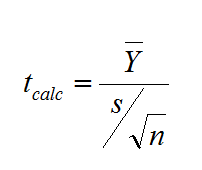## One Sample t Test with SigmaXL

What is a One Sample t Test? A One sample t test is a hypothesis test to study whether there is a statistically significant difference between a population mean and a specified value. Where: What is a t-Test? In statistics, a t-test is a hypothesis test in which the test statistic follows a Student’s t […]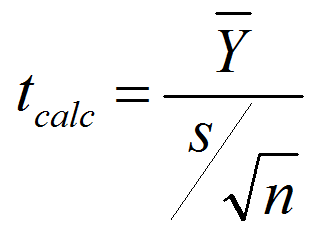## One Sample t Test with Minitab

What is a t Test? In statistics, a t test is a hypothesis test in which the test statistic follows a Student’s t distribution if the null hypothesis is true. We apply a one sample t test when the population variance (σ) is unknown and we use the sample standard deviation (s) instead. A hypothesis […]## One Sample t Test with JMP

One Sample t Test What is a t Test? In statistics, a t test is a hypothesis test in which the test statistic follows a Student’s t distribution if the null hypothesis is true. We apply a one sample t test when the population variance (σ) is unknown and we use the sample standard deviation […]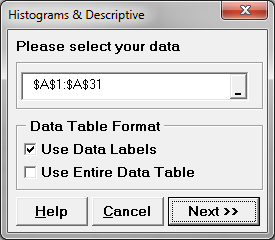## Central Limit Theorem with SigmaXL

Central Limit Theorem The Central Limit Theorem is one of the fundamental theorems of probability theory. It states a condition under which the mean of a large number of independent and identically-distributed random variables, each of which has a finite mean and variance, would be approximately normally distributed. Let us assume Y1, Y2 . . […]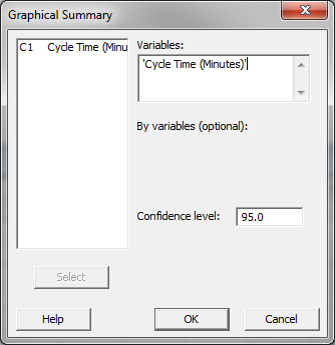## Central Limit Theorem with Minitab

What is Central Limit Theorem? The Central Limit Theorem is one of the fundamental theorems of probability theory. It states a condition under which the mean of a large number of independent and identically-distributed random variables, each of which has a finite mean and variance, would be approximately normally distributed. Let us assume Y1, Y2 […]## Central Limit Theorem with JMP

What is Central Limit Theorem? The Central Limit Theorem is one of the fundamental theorems of probability theory. It states a condition under which the mean of a large number of independent and identically-distributed random variables, each of which has a finite mean and variance, would be approximately normally distributed. Let us assume Y1, Y2 […]## Multi Vari Analysis with SigmaXL

Multi-Vari Analysis Multi Vari Analysis is a graphic-driven method to analyze the effects of categorical inputs on a continuous output. It studies how the variation in the output changes across different inputs and helps us quantitatively determine the major source of variability in the output. Multi Vari charts are used to visualize the source of […]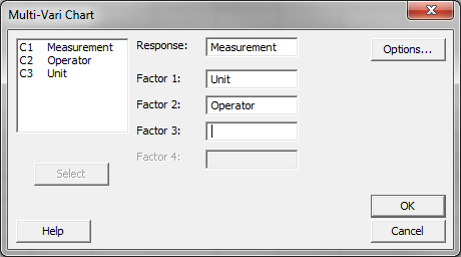## Multi Vari Analysis with Minitab

What is Multi Vari Analysis? Multi Vari analysis is a graphic-driven method to analyze the effects of categorical inputs on a continuous output. It studies how the variation in the output changes across different inputs and helps us quantitatively determine the major source of variability in the output. Multi-Vari charts are used to visualize the […]## Multi Vari Analysis with JMP

What is Multi-Vari Analysis? Multi vari analysis is a graphic-driven method to analyze the effects of categorical inputs on a continuous output. It studies how the variation in the output changes across different inputs and helps us quantitatively determine the major source of variability in the output. Multi-vari charts are used to visualize the source […]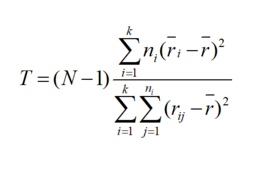## Kruskal Wallis with SigmaXL

Kruskal–Wallis One-Way Analysis of Variance The Kruskal Wallis one-way analysis of variance is a statistical hypothesis test to compare the medians among more than two groups. It is an extension of Mann–Whitney test. While the Mann–Whitney test allows us to compare the samples of two populations, the Kruskal–Wallis test allows us to compare the samples […]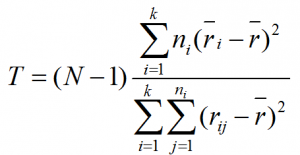## Kruskal Wallis with Minitab

Kruskal Wallis One-Way Analysis of Variance The Kruskal Wallis one-way analysis of variance is a statistical hypothesis test to compare the medians among more than two groups. It is an extension of Mann–Whitney test. While the Mann–Whitney test allows us to compare the samples of two populations, the Kruskal–Wallis test allows us to compare the […]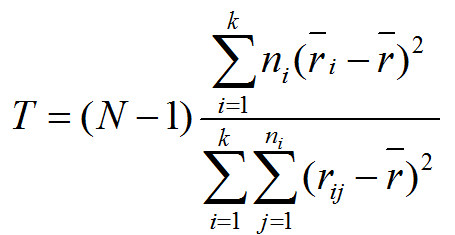## Kruskal Wallis with JMP

What is Kruskal–Wallis One-Way Analysis of Variance? The Kruskal Wallis one-way analysis of variance is a statistical hypothesis test to compare the medians among more than two groups. It is an extension of Mann–Whitney test. While the Mann–Whitney test allows us to compare the samples of two populations, the Kruskal Wallis test allows us to […]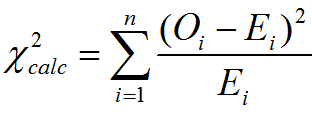## Chi Square Test with JMP

Chi Square (Contingency Tables) We have looked at hypothesis tests to analyze the proportion of one population vs. a specified value, and the proportions of two populations, but what do we do if we want to analyze more than two populations? A chi-square test is a hypothesis test in which the sampling distribution of the […]## Chi Square Test with SigmaXL

Chi Square (Contingency Tables) We have looked at hypothesis tests to analyze the proportion of one population vs. a specified value, and the proportions of two populations, but what do we do if we want to analyze more than two populations? A chi-square test is a hypothesis test in which the sampling distribution of the […]## Chi Square Test with Minitab

Chi Square (Contingency Tables) We have looked at hypothesis tests to analyze the proportion of one population vs. a specified value, and the proportions of two populations, but what do we do if we want to analyze more than two populations? A chi-square test is a hypothesis test in which the sampling distribution of the […]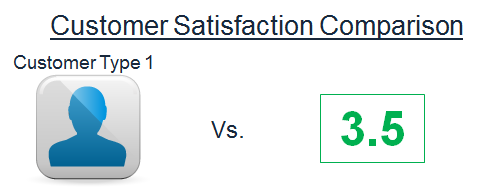## One Sample Wilcoxon Test with Minitab

What is the One Sample Wilcoxon Test? The one sample Wilcoxon test is a hypothesis test to compare the median of one population with a specified value. Null Hypothesis (H0): η = η0 Alternative Hypothesis (Ha): η ≠ η0 It is an alternative test of one sample t-test when the distribution of the data is non-normal. It is [...]## One Sample Wilcoxon Test with SigmaXL

What is the One Sample Wilcoxon Test? The one sample Wilcoxon test is a hypothesis test to compare the median of one population with a specified value. Null Hypothesis (H0): η = η0 Alternative Hypothesis (Ha): η ≠ η0 It is an alternative test of one sample t-test when the distribution of the data is non-normal. It is [...]## One Sample Wilcoxon Test with JMP

What is the One Sample Wilcoxon Test? The one sample Wilcoxon test is a hypothesis test to compare the median of one population with a specified value. Null Hypothesis (H0): η = η0 Alternative Hypothesis (Ha): η ≠ η0 It is an alternative test of one sample t-test when the distribution of the data is non-normal. It is [...]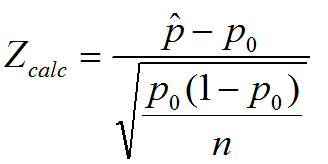## One Sample Proportion with JMP

What is the One Sample Proportion Test? One sample proportion test is a hypothesis test to compare the proportion of one certain outcome (e.g. the number of successes per the number of trials, or the number of defects per the total number of opportunities) occurring in a population following the binomial distribution with a specified [...]## One Sample Proportion with Minitab

What is the One Sample Proportion Test? One sample proportion test is a hypothesis test to compare the proportion of one certain outcome (e.g. the number of successes per the number of trials, or the number of defects per the total number of opportunities) occurring in a population following the binomial distribution with a specified [...]